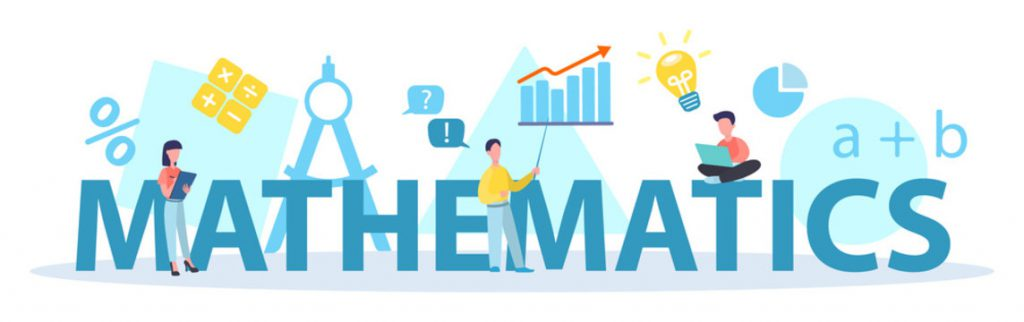Math needs practice, interest and brainpower which are fostered by the Math lab. The D.A.V. Alok Public School Math Lab makes Math simple and fun for students. The lab is well equipped to demonstrate and elucidate any topic. For instance, the ‘RAMANUJAN’S KIT’ has all the components with which every important concept and formula can be proved practically. It also enables the teachers to demonstrate and reinforce Mathematical concepts. Hence, it helps in mental enhancement of students in any area of Math like Algebra, Arithmetic, Calculus, Geometry, Statistics, and Trigonometry etc.Departmental library in the lab helps students to browse through the books on various topics.